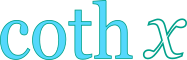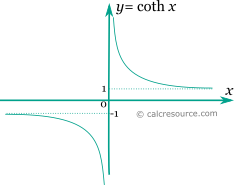Hyperbolic cotangent calculator

This tool evaluates the hyperbolic cotangent of a number: coth(x). Enter the argument x below.

 x = Result: coth(x) =Definitions

General

The hyperbolic cotangent function is defined as:

The graph of the hyperbolic cotangent function is shown in the figure below. Unlike the trigonometric cotangent, the function is not periodic.Series

All hyperbolic functions can be defined in an infinite series form. Hyperbolic cotangent function can be written as:

The above series converges for . Bndenotes the n-th Bernulli number .

Properties

The derivative of the hyperbolic cotangent function is:

The integral of the hyperbolic cotangent is given by: